# 3076 Exam¶

Semester 1 2016

**CONFIDENTIAL**

SID:

SEAT NUMBER:

SURNAME:

Other Names:

Lecturer: S. Olver

June 2015

Time allowed: Two hours

**This paper is CONFIDENTIAL.**
**Only the course website may be accessed:**
http://www.maths.usyd.edu.au/u/olver/teaching/MATH3976/
**In addition, do NOT access any files on the machine, either inside or outside your user directory.**

This paper is worth 60% of the maximum mark for this course.

The questions are marked with an A, B or C. To earn a score of 65/100, complete all C questions. To earn a score of 85/100, complete all B and C questions. To earn a score of 100/100, complete all A, B and C questions.

If not all questions are completed, A questions will be weighted more than B questions, which will be weighted more than C questions.

All answers to questions must be entered in one or more cells immediatly below each question, and preceding the following question.

Default cells are provided below each question. If desired, additional cells can be added by clicking the + button in the toolbar.

The answers should be given as working Julia code. You are allowed to use all inbuilt Julia routines, as well as those in the included packages (ODE and PyPlot).

Pseudo-code, non-working answers and textual explanations will recieve partial credit. Please comment your code so that you may recieve partial credit for wrong or incomplete answers.

Any questions that do not ask for code can be answered in comments, or as a separate plain text cell. You can make a cell plain text by selecting NBConvert from the drop-down menu that says Code, after the ⟳ button. Try changing the following cell to NBConvert:

In [ ]:
This is a bit of plain text explaining the solution to the code, because I couldn't figure out how to answer the question in Julia.  I don't want it to be parsed as Julia as it will give an error message, and not wrap correctly.


It may be useful to restart the kernel between questions, by clicking the ⟳ button. Otherwise, you have issues arising from redefining variables as functions if you reuse the variable/function name.

Before we begin, test that the following 3 boxes of Julia commands are working correctly:

In :
v=[1,3,2]
v+3

Out:
3-element Array{Int64,1}:
4
6
5
In :
using PyPlot
plot(1:10,exp(1:10))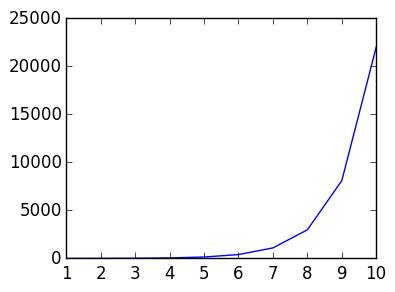Out:
1-element Array{Any,1}:
PyObject <matplotlib.lines.Line2D object at 0x312e75b90>
In :
using ODE
t,y=ode45((t,y)->y*(1-y),0.5,0.:.1:10.)
plot(t,y)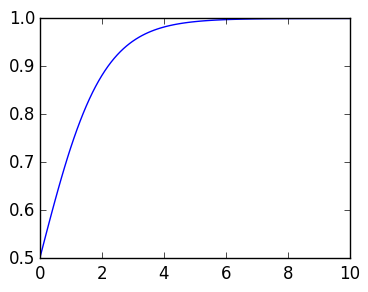Out:
1-element Array{Any,1}:
PyObject <matplotlib.lines.Line2D object at 0x3130f8590>

# DO NOT SCROLL PAST THIS POINT UNTIL THE START OF THE EXAM READING TIME¶

## Strings¶

Question 1 (C) Complete the following function that creates a new string consisting of the characters of a, followed by "hi", followed by the characters of b:

# INPUT: two strings a and b
# OUTPUT: a string
function histring(a::AbstractString,b::AbstractString)
# TODO: concatenate a, "hi", and b
end

In :
function histring(a::AbstractString,b::AbstractString)
a*"hi"*b
end

Out:
histring (generic function with 1 method)

## Floating point arithmetic¶

Question 2 (C)

1. What is the smallest normal Float64?
2. What are its bits?
In :
x=realmin(Float64)
bits(realmin(Float64))

Out:
"0000000000010000000000000000000000000000000000000000000000000000"

## Vectors and matrices¶

Question 3 (C) Complete the function

#INPUT: an Integer n
#OUTPUT: A Vector{Float64}
function primevector(n::Integer)
#TODO: create a zero vector v of length n, set prime entries to 1, and return v
end


that returns a vector of length n whose prime entries are equal to 1, and non-prime entries are equal to zero.

Hint: isprime(x) returns whether a number is prime or not.

In :
#INPUT: an Integer n
#OUTPUT: A Vector{Float64}
function primevector(n::Integer)
v=zeros(n)
for k=1:n
if isprime(k)
v[k]=1
end
end
v
end

primevector(24)

Out:
24-element Array{Float64,1}:
0.0
1.0
1.0
0.0
1.0
0.0
1.0
0.0
0.0
0.0
1.0
0.0
1.0
0.0
0.0
0.0
1.0
0.0
1.0
0.0
0.0
0.0
1.0
0.0

## Linear Algebra¶

Question 4 (C) Calculate the vector x that solves A*x=b, for

A=[1  2  3 ;
4  5  6 ;
7  8  10]
b=[1,2,3]

In :
A=[1  2  3 ;
4  5  6 ;
7  8  10 ]
b=[1,2,3]
x=A\b

Out:
3-element Array{Float64,1}:
-0.333333
0.666667
0.0     

Question 5 (B) For the matrix A above, use the svd of A to solve A*x=b, without using \.

In :
U,σ,V=svd(A)

v=U'*b

for k=1:3
v[k]=v[k]/σ[k]
end

V*v

Out:
3-element Array{Float64,1}:
-0.333333
0.666667
-2.83107e-15

## Condition numbers and Error Analysis¶

Question 6 (C) What is the worst case relative error of ${\rm fl}(x)$, where $x$ is a real number and ${\rm fl}$ is the function that a real number to the closes Float64?

In :
eps(Float64)/2

Out:
1.1102230246251565e-16

Question 7 (B)

1. Calculate numerically the relative forward error in approximating a single step of Newton iteration for $x^2 - 2 = 0$: $$x_k - {x_k^2 -2 \over 2 x_k}$$ By its Float32 calculation $$x_k \ominus ((x_k \otimes x_k -2) \oslash (2x_k)),$$ where $x_k=3$. You can assume $x_k$ is given as a Float32 and treat Float64 calculations as exact.

2. Why is the use of $2x_k$ instead of $2 \otimes x_k$ and $a-2$ instead of $a \ominus 2$ correct?

In :
xk=3f0
(xk-(xk^2-2)/(2xk)-1.8333333333333333)/1.8333333333333333

Out:
2.1674416322331975e-8
In :
xk=3
xk-(xk^2-2)/(2xk)

Out:
1.8333333333333333

Question 8 (C)

1. Complete the function

## INPUT: a function f and an integer n
## OUTPUT: a Float64 approximating the integral of f using the lhrule rule
function lhrule(f::Function,n::Integer)
#TODO: return the lefthand rule to approximate
end


that implements the lefthand rule, so that $$\int_0^1 f(x) dx \approx {1 \over n} \sum_{k=0}^{n-1} f(x_k)$$ where $x_k \triangleq kh$ for $h=1/n$.

2. Show that the method approximates $\int_0^1 e^{x} dx$ for $n=10000$ with an error less than 1E-4.

In :
## INPUT: a function f and an integer n
## OUTPUT: a Float64 approximating the integral of f using the midpoint rule

function lhrule(f::Function,n::Integer)
sum(map(f,linspace(0.,1.-1/n,n)))/n
end

Out:
lhrule (generic function with 1 method)
In :
lhrule(x->exp(x),10000)-(exp(1.)-1.)

Out:
-8.591265952118121e-5

Question 9 (B)

1. Estimate the rate of convergence for lhrule approximating $\int_0^1 e^x dx$ by finding an $\alpha$ so that the error decays like $Cn^\alpha$.
2. How does this rate of convergence compare to the right-hand rule and Trapezium rule?

Hint: it may help to do a loglog plot.

In :
ns=round(Int,logspace(1,5,10))

err=zeros(length(ns))
for k=1:length(ns)
err[k]=abs(lhrule(x->exp(x),ns[k])-(exp(1.)-1.))
end

using PyPlot
loglog(ns,err)
loglog(ns,ns.^(-1.0))

# This is O(n^{-1}), matching the  the right-hand rule O(n^{-1}) and worse than the trapezium rule O(n^{-2})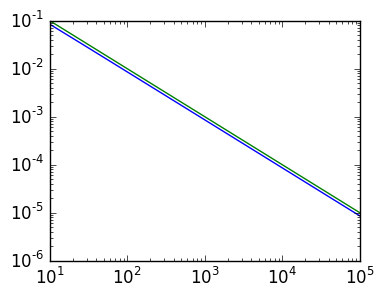Out:
1-element Array{Any,1}:
PyObject <matplotlib.lines.Line2D object at 0x30ab57690>

Question 10 (A)

1. Consider the function $$f(x)=x^2(1-x)^2exp(x).$$
Give a formula for the asymptotic behaviour (not bound!) of the error in approximating by the Trapezoidal rule. That is, find a $C$ and $\alpha$ so that $$\int_0^1 f(x) dx- h \left({f(0) \over 2} + \sum_{k=1}^{n-1} f(x_k) + {f(1) \over 2}\right) \sim C n^{\alpha}.$$

2. Demonstrate that your expression is correct using a plot to show that the relative error tends to zero. You can use any inbuilt routines to determine the exact integral.

Hint: You can use the following routine from lectures:

function trap(f,n)
h=1/n
x=linspace(0,1,n+1)

v=map(f,x)
h/2*v+sum(v[2:end-1]*h)+h/2*v[end]
end

In :
# It has two derivatives vanishing

f = x->x^2*(1-x)^2*exp(x)

# (1-x)^2 == 1-2x+x^2

#f=(x^2-2x^3+x^4)*exp(x)

#f'  = (2x-6x^2+4x^3 +x^2-2x^3+x^4)exp(x)
#f'  = (2x-5x^2+2x^3+x^4)exp(x)
#f'' = (2-10x+6x^2+4x^3+2x-5x^2+2x^3+x^4)exp(x)
#f'' = (2-8x+x^2+6x^3+x^4)exp(x)
#f''' = (-8+2x+18x^2+4x^3+2-8x+x^2+6x^3+x^4)exp(x)
#f''' = (-8+2x+18x^2+4x^3+2-8x+x^2+6x^3+x^4)exp(x)

function trap(f,n)
h=1/n
x=linspace(0,1,n+1)

v=map(f,x)
h/2*v+sum(v[2:end-1]*h)+h/2*v[end]
end
fppp=x->(-8+2x+18x^2+4x^3+2-8x+x^2+6x^3+x^4)*exp(x)

ns=1:100
err=zeros(length(ns))

for n=ns
err[n]=(trap(f,n)-ex+(fppp(1)-fppp(0))/(30factorial(4)*n^4))/(trap(f,n)-ex)
end

plot(abs(err))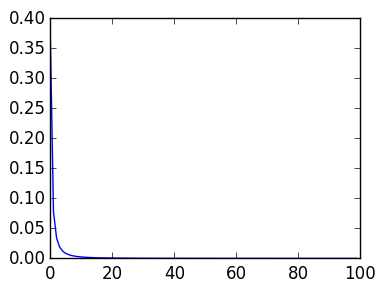Out:
1-element Array{Any,1}:
PyObject <matplotlib.lines.Line2D object at 0x30aa1a2d0>

## The Discrete Fourier Transform¶

Question 11 (B) Consider the even function $f(\theta) = \cos({\cos \theta})$.

1. Calculate coefficients $c_k$ so that $$f(\theta) \approx \sum_{k=0}^5 c_k \cos k \theta.$$
2. Show that the approximation has an error close to 2E-6 when evaluating at $\theta=0.6$.

Hint: Remember that you can use any inbuilt routines, or the trap function above.

In :
f=θ->cos(cos(θ))

n=5
c=zeros(n+1)
for k=0:n
end

c/=2

ret=0.
for k=0:n
ret+=cos(0.6k)*c[k+1]
end
ret-f(0.6)

Out:
-3.75692460103938e-5

## ODE and PDE Solving¶

Question 12 (C)

1. Approximate numerically

$$y'(t) = (1-y(t))y(t), y(0)=0.5$$
for $0 \leq t \leq 1.$
2. Plot the approximate $y$.

Hint: Remeber that you can use any inbuilt ODE solver.

In :
## [y,y'] == [y'; t^2 y]

using ODE
t,y=ode45((t,y)->(1-y)*y,0.5,0.:0.01:1.)

plot(t,y)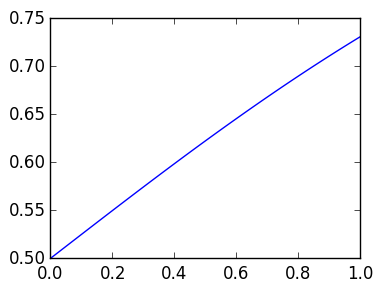Out:
1-element Array{Any,1}:
PyObject <matplotlib.lines.Line2D object at 0x309cc79d0>

Question 13 (A)

1. Approximate the solution the heat equation
$$u_t = u_x + u_{xx}$$

with Dirichlet boundary conditions $u(0) = u(1) = 0$, using Backward Euler in time and the initial condition $u_0(x)=x(1-x)exp(x)$. Plot the approximate solution at time $t = 1$. Use n=100, $\Delta x=1/n$ and $\Delta y$ sufficiently small so that the approximation is stable.

1. Plot the approximate solution at time $t = 1$.

It may help to use the following functions from lectures

function D(h,n)
ret=zeros(n,n+1)
for k=1:n
ret[k,k]=-1/h
ret[k,k+1]=1/h
end
ret
end

In :
function D(h,n)
ret=zeros(n,n+1)
for k=1:n
ret[k,k]=-1/h
ret[k,k+1]=1/h
end
ret
end

n=100
Δx=1/n
Δt=Δx^2/2

B0=[1 zeros(1,n)]
B1=[zeros(1,n) 1]

D2=D(Δx,n-1)*D(Δx,n)
DD=D(Δx,n)[1:n-1,:]

x=linspace(0.,1.,n+1)
u0=x.*(1-x).*exp(x)

II=eye(n+1)[2:end-1,:]

u=u0

for k=1:1/Δt
u=[B0;II-Δt*(D2+DD);B1]\u
end

In :
plot(u)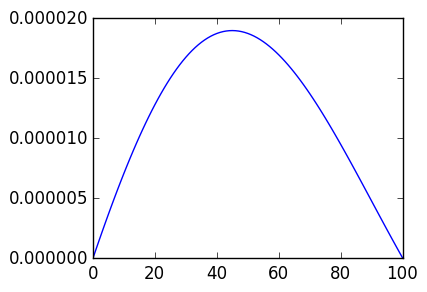Out:
1-element Array{Any,1}:
PyObject <matplotlib.lines.Line2D object at 0x30bf87a90>
In :
function D(h,n)
ret=zeros(n,n+1)
for k=1:n
ret[k,k]=-1/h
ret[k,k+1]=1/h
end
ret
end

n=100
Δx=1/n
Δt=Δx^2/2

B0=[1 zeros(1,n)]
B1=[zeros(1,n) 1]

D2=D(Δx,n-1)*D(Δx,n)
DD=D(Δx,n)[2:n,:]

x=linspace(0.,1.,n+1)
u0=x.*(1-x).*exp(x)

II=eye(n+1)[2:end-1,:]

u=u0

for k=1:1/Δt
u=[B0;II-Δt*(D2+DD);B1]\u
end

In :
plot(u)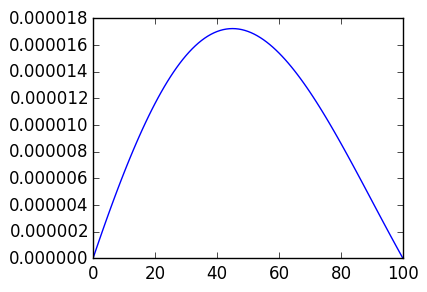Out:
1-element Array{Any,1}:
PyObject <matplotlib.lines.Line2D object at 0x30c0abed0>
In [ ]: